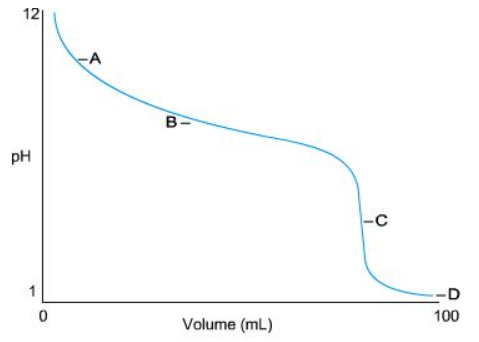# Identify the equivalence point on the titration curve shown hereIdentify the equivalence point on the titration curve shown here. A is the equivalence point B is the equivalence paint C is the equivalence point pH D is the equivalence pointDefine the end point of a titration. It is the point at which the pH no longer changes. It is when a change that indicates equivalence is observed in the analyte solution. It is a synonym for equivalence point.

General guidance

Concepts and reason
This is a problem based on titration curve showing a titration between an acid and a base so as to identify the equivalence point.
In a titration, the point where the amount of titrant added neutralizes the analyte solution completely is the equivalence point.
The point at which an indicator changes color is the end point.

Fundamentals

Titration curve is a plot of the pH as a function of added titrant. At a certain point in a titration of a strong acid or base analyte against the titrant (base or acid), the number of moles of analyte becomes equal to the number of moles of the titrant. This point is called the equivalence point. At this point there is very large change in pH over a small range of the added titrate volume .
Equivalence point is where the titration curve exhibits an inflection point.

Step-by-step

Step 1 of 4

From the figure, the points A, B and D are not the equivalence points.

The points A, B and D are not the equivalence points because they do not exhibit a large change in pH and are not steep slopes.

Step 2 of 4

From the figure, C is the steepest point and hence it is the equivalence point.

The equivalence point in the given curve is C.

From the curve, we come to know that the titration was started at pH 12 of the analyte. When the volume of titrant is increased the pH is decreased gradually. This can be observed by the shape of curve around points A and B.
But near point C, there is a large change in the pH with a small change in the volume.
The steep slope can be seen at point C in the curve.
Thus C is the equivalence point.

It is important to carefully observe the slope of the titration curve.

Step 3 of 4

End point is not defined as the point at which pH no longer changes or a synonym for equivalence point.

End point of a titration comes after equivalence point. Till the equivalence point the indicator color does not change. The indicator changes its color after equivalence point.

Step 4 of 4

The correct definition for end point is that end point is the change that indicates equivalence is observed in the analyte solution.

It is when a change that indicates equivalence is observed in the analyte solution.

The end point of a titration comes after the equivalence point when the medium of the titration is changed and the indicator changes its color. At end point, the concentration of titrant exceeds the concentration of titrating mixture.

The equivalence point in the given curve is C.

It is when a change that indicates equivalence is observed in the analyte solution.